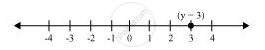# Give the geometric representations of y = 3 as an equation in one variable. - Mathematics

Give the geometric representations of y = 3 as an equation in one variable.

#### Solution

In one variable, y = 3 represents a point as shown in following figure.Concept: Equations of Lines Parallel to the X-axis and Y-axis
Is there an error in this question or solution?

#### APPEARS IN

NCERT Class 9 Maths
Chapter 4 Linear Equations in two Variables
Exercise 4.4 | Q 1.1 | Page 77